# Deep Learning for Natural Image Segmentation Priors

## Gabriel Hope

May 10, 2016

Image segmentation is an important problem in computer vision. Distinguishing different objects and regions within an image is an extreamly useful preprocessing step in applications that require full scene understanding as well as many applications for image processing and editing. There are several different ways to frame the segmentation problem in computer vision. For example, semantic segmentation is a common segmentation task that can viewed as an extension of object detection. In the semantic segmentation task, rather than finding bounding box for objects of a specified class, the goal is to find the boundaries of a target object at the pixel level.

In this project, I look at the more general segmentation task, where the goal is to partition an image into regions that correspond to different objects or materials in the image. Correctness in this setting is often difficult to define precisely, as objects boundaries are often ill-defined (should a t-shirt be considered the same object as the person who is wearing it?). In this case, a common proxy for "truth" data are human-generated segmentations of images.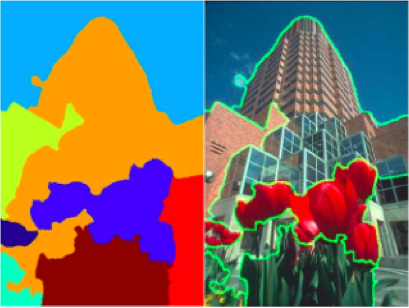Above: An example of a "good" general segmentation for an image (from ).

Many techniques have been proposed for the image segmentation task. Common examples include simple color-based K-means, graph-cutting approaches and Markov random fields . The work in this project is based on the Spatially Dependent Pitman-Yor model from Sudderth and Jordan  (and extended by Ghosh and Sudderth ) that extends nonparametric mixture models to the segmentation problem. In this project I extend this segmentation model to make use of large amounts of labeled image data by incorporating the output of deep networks into the prior distribution on image segmentations.

## Nonparametric Segmentation

I will first give a brief, high-level overview of the spatially dependent Pitman-Yor proecss model for segmentation. This model uses a layered approach to segmentation, where segments are intuitively considered as overlapping layers rather than strictly adjacent regions.

An easy way to understand the Spatially dependent Pitman-Yor model is to first treat it as a foreground-background segmentation model. In this context, the model assumes that images are generated using the following process:

• First an assignment surface is randomly drawn for the image. This surface is drawn from a zero-mean Gaussian process and every point on the image has a corresponding point on the surface. In practice, this surface is represented by a "height" value for each pixel of the image. A draw of a Gaussian process over a finite number of points is equivalent to a draw from a multivariate Gaussian (normal) distribution, so in practice the heights for all the pixels are drawn jointly from a zero-mean multivariate Gaussian distribution. Determining what to use for the between-pixel covariances in this distribution is the fundamental problem explored in this project.
• Next a random threshold value is drawn from a standard normal distribution (Or this threshold is set to 0).
• Pixels whose height on the assignment surface are above the threshold are assigned to the forground segment, while all other pixels are assigned to the background.
• Each segment is given a different random distribution (such as a 3-d normal distribution) and the pixels in each segment are drawn from the corresponding distribution.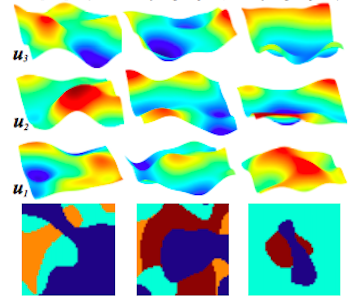Above: An illustration of assignment surfaces for different layers and the resulting segmentations (from ).

The process above defines a distribution over possible segmentations and possible images. With a given image, the actual pixels are observed and it is possible to use variational inference to find an approximate posterior distribution for segmentation conditioned on the observed pixels. This is the technique that I used to generate the segmentations in this project.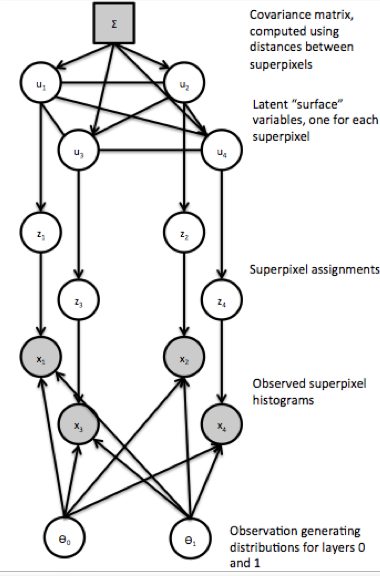Above: The graphical model for a simplified version of the foreground-background model where the threshold is fixed at 0.

Extending this model to nonparametric segmentation (segmentation with an abitrary number of segments) is straightforward. The generative process is simply repeated so that pixels assigned the forground are further segmented using the same process. This process can be repeated until there are no more pixels left in the foreground to further segment. In this case the threshold distribution is modified so that the number of segments roughly follows a power-law distribution.

It's important to note that this model does not acutally work on the pixels of the image directly. Instead, as a preprocessing step, the image is dived into 1000 superpixels using the SLIC algorithm . Each superpixel is represented by a 125-bin histogram of the colors in the superpixel as well as an 128-bin histogram of texton assignments. A texton is a cluster of responses from a set of texture filters. Each pixel in a given image is assigned a texton by first applying the set of filters centered at its location, then assigning the vector of responses to the nearest texton cluster.

Because the superpixels are represented as histograms, the segment-specific distributions that generate them are assumed to be multinomial distributions, which in turn are drawn from a shared Dirichlet distribution.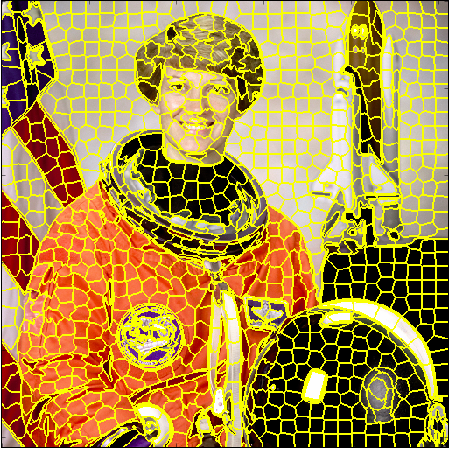Above: An image and the corresponding SLIC superpixel segmentation.

## Data

For this project, I used the Berkeley Image Segmentation dataset  (BSDS300) for evaluating segmentations and in some cases for training. This dataset consists of 300 natural photographs of a diverse set of scenes. The advantage of using this dataset is that it provides multiple human segmentations of each of the images that can be used as a proxy for ground truth segmentaions. Some examples from this dataset are shown below. Because computing each segmentation with the spatially dependent Pitman-Yor model is currently very slow, I only actually evaluted using a subset of 10 of the BSDS images.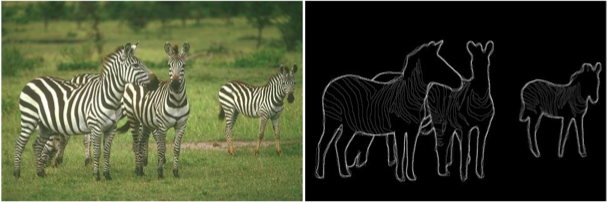Above: An example image from the BSDS dataset along with the corresponding human segmentation(s).

## Learning Segmentation Priors

One can modify the segmentation prior for a given image by modifying the assignment surface covariances between pixels in the image. Intuitively, the covariance between two pixels should be high if the pixels are likely to be part of the same segment and low if they are likely to be part of different segments.

The simplest approach to generating pixelwise covariances is to base the covariances on the distance between pixels so that pixels that are closer in the image have a higher covariance. In order to generate distance-based covariance between two superpixels, I took the mean of the coordiates of each superpixel and applied a Gaussian kernel to the difference as a distance measure. This approach creates covariances between 0 and 1, where pixels that are very close will have a covariance close to 1 and pixels that are very apart far will have covariance close to zero. The kernel width (gamma) was chosen by eye and is set to 0.005.

The core of this project is to use a data-driven approach to generating appropriate pixelwise covariances for the segmentation model. My general approach to this problem was to use existing CNNs trained on large, labeled datasets to generate some kind of prediction for each pixel in an image. These predictions are then incorporated into the pixelwise covariances to improve them over the baseline distance-based covariances.

The first network that I used in the project was a fully convolutional network for semantic segmentation from Long et. al . This network was trained for the semantic segmentation task on the Pascal VOC 2012 dataset. For a given image, this network outputs a 60-class (including background) probability vector at each pixel.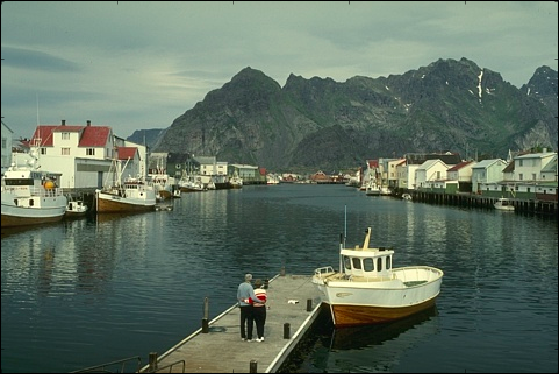Above: An example image from BSDS.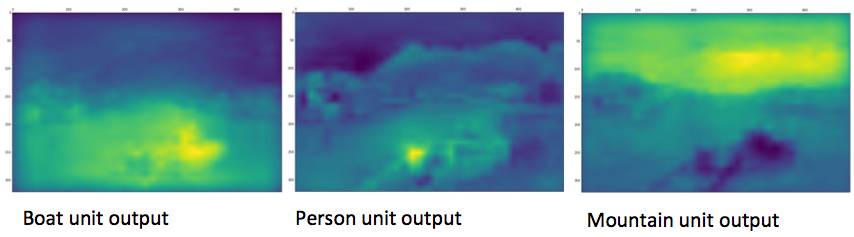Above: CNN predicted class probabilities for three different classes on the example image.

The second network that I applied to this task was the depth map prediction network from Eigen et. al . This network was trained on the NYU Depth dataset and it outputs a predicted depth at each pixel in the image. Its output is designed to approximate the output of a standard depth sensor like a Microsoft Kinect.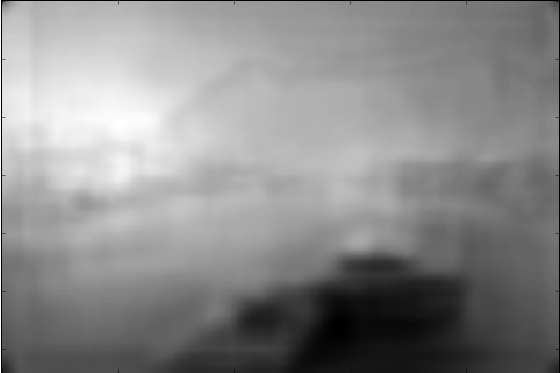Above: CNN predicted depth map for the example image.

I considered two different approaches to incorporate the outputs of these networks into the superpixel covarianaces for each image. The first approach that I used was to simply take the mean network output for each superpixel and concatenate this output with the mean pixel location for each superpixel to create a feature vector for each superpixel (rescaling the nework output to match the scale of the pixel locations). To compute the covariance between two pixels, I then simply used the same Gaussian kernel distance as before.Above: An example segmentation using the depth-inclusive prior.

For the second approach, I wanted to learn a relative weighting for each type of feature (pixel locations, semantic segmentation outputs and depth). In this case I created a feature for each pair of superpixels by concatenating the euclidean distance between the locations, the euclidean distance between the semantic segmentation output vectors and the absolute difference between the depth predictions. I then took a subsample of 500 pairs of superpixels from each of 30 random images from the BSDS dataset and trained a logistic regression classifier to predict the probability that two superpixel are part of the same segement given a vector of feature distances. The truth labels for training were taken from the human segmentations of the BSDS dataset. This approach was inspired by the Ghosh and Sudderth paper , which also describes a technique for translating the probability that two pixels are part of the same segment into an appropriate covariance.

## Evaluation

The results of segmentations can be subjective, so evalutaing by generated segmentations by eye is still one of the best ways to understand the performance of a segmentation algorithm. In addition to evaluating by eye, I also used the Probibalistic Rand Index  measure to evaluate segmentation using the BSDS human segmentations as ground truth. This is an extension to the Rand index measure for comparing clusterings that is better suited to evaluating image segmentations. The basic Rand index is defined as the number of element pairs that are correctly identified as being part of the same group or not divided by the total number of possible element pairs in the data. A Rand index will be between 0 and 1 and a higher index is better.

## Results

The table below summarizes the probibalistic rand index evaluation of the segmentation model under different priors, averaged over all human segmentations for the images in my test set.

Prior Average PRI
Pixel Distances 0.672
Pixel Distances + Semantic Output 0.695
Pixel Distances + Depth 0.683
Pixel Distances + Semantic Output (Learned Weighting) 0.526
Pixel Distances + Depth (Learned Weighting) 0.691
Pixel Distances + Semantic + Depth (Learned Weighting) 0.5912

According to this measure the best segmentation prior is the one that incorporates the output of the semantic segmentation network naively (instead of learning the relative weighting between features).

Below are the segmentations from all 6 versions of the model on 2 sample images, one of the better examples and one of the worse ones.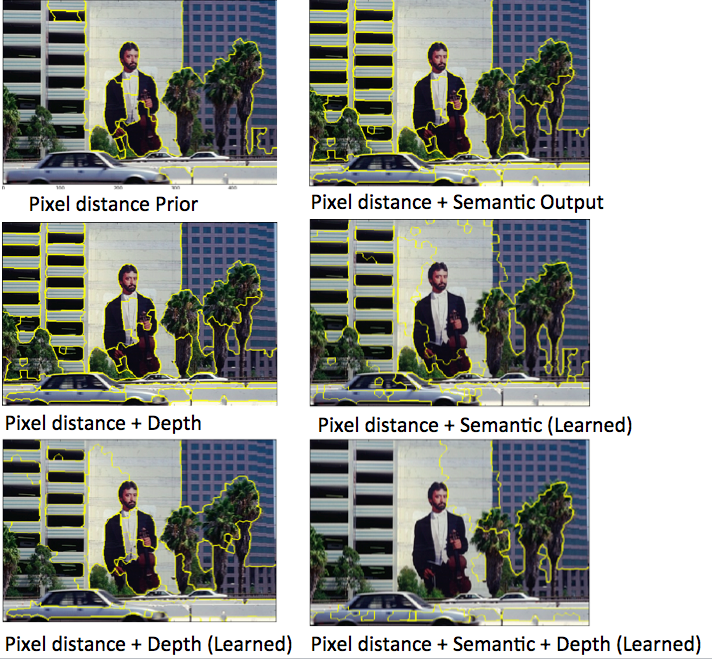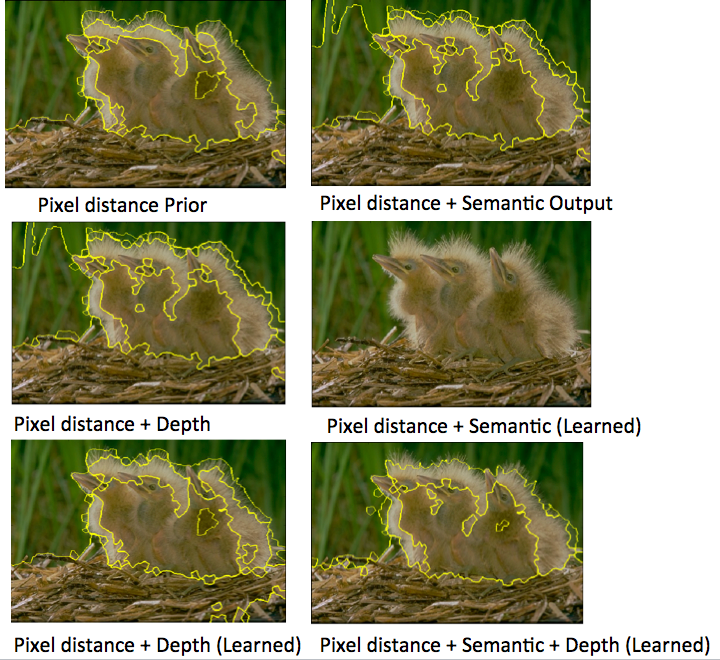## Future Work

The most straighforward line of future work would be to replace the semantic segmentation or depth network that I used here with a network fine-tuned to the task of predicting pairwise pixel covariances. This is likely a difficult task that would require a vast number of general human segmentations. It could conceivably be accomplished by designing a network that maps an image into an alternate colorspace such that color distances can be mapped to covariances.

Another important point of weakness in the spatially dependent Pitman-Yor process model is the representation of superpixels. As I described above, superpixels are represented as histograms of colors and texture responses across the contained pixels. This is a fairly naive representation for superpixels which may not be all that good for distinguishing object boundaries. By the nature of these features, two quite different looking superpixels could have similar representations. The representation also doesn't account for context around a superpixel and similarly doesn't account well for cases when different looking superpixels are still commonly part of the same object.

A better approach to representation could be to learn representations for superpixels, possibly using convolutional neural networks. An approach in this vein would require a significant amount of additional research. As a first step, I explored replacing the texture filters used in the standard model with filters from a trained classification CNN to see if they might create features that better discriminate between different object types.

The images below show the texture feature assignments of normal texture features across a sample image compared to the assignments using filters learned as the first layer output of the AlexNet CNN. While the CNN-filter responses appear to be more diverse, which could allow them to be more discriminative, the resulting segmentations are indistinguishable for this example.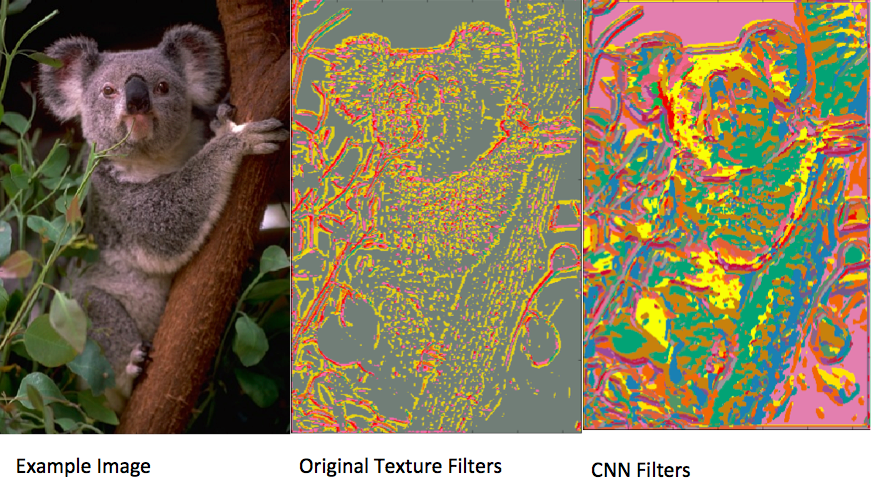## Discussion

Ultimately, the results of this project are somewhat underwhelming. While the results show that incorporating the network ouputs can improve segmentations somewhat, the difference is very small and the small number of images that the final models were tested on means that the difference may not actually be significant. Still, it is possible that with more fine-tuning of both the pipline and the model settings, that my approach could make a more significant differnce. I intend to trying the ideas explored here in my future work on segmentation. I am particularly interested in the results of the model that incorporates estimated depth, as this model does not rely on specfic object types and could theoretically be applied to any scene regardless of content.

The quality of the results was largely hampered by a number of issues the I encountered during the course of this project. Mainly these issues stemmed from the segmentation model itself. Inference for the spatially dependent Pitman-Yor process model is complex and the results can be dependent on how the algorithm is intialized, which can make comparing various tweaks to the model quite difficult. Furthermore, the code for inference is still unstable, under development (by me) and extremely slow. This made debugging difficult and made trying to fine-tune the segmentation pipeline an arduous process. Beacause iterating on changes the the priors and the model was so slow, many of the settings I used to generate the final results relied on guesswork rather than a pricipled comparison.

## References

 Sudderth, Erik B., and Michael I. Jordan. "Shared segmentation of natural scenes using dependent Pitman-Yor processes." Advances in Neural Information Processing Systems. 2009.

 Ghosh, Soumya, and Erik B. Sudderth. "Nonparametric learning for layered segmentation of natural images." Computer Vision and Pattern Recognition (CVPR), 2012 IEEE Conference on. IEEE, 2012.

 Unnikrishnan, Ranjith, and Martial Hebert. "Measures of similarity." Application of Computer Vision, 2005. WACV/MOTIONS'05 Volume 1. Seventh IEEE Workshops on. Vol. 1. IEEE, 2005.

 Eigen, David, Christian Puhrsch, and Rob Fergus. "Depth map prediction from a single image using a multi-scale deep network." Advances in neural information processing systems. 2014.

 Achanta, Radhakrishna, et al. "SLIC superpixels compared to state-of-the-art superpixel methods." Pattern Analysis and Machine Intelligence, IEEE Transactions on 34.11 (2012): 2274-2282.

 Long, Jonathan, Evan Shelhamer, and Trevor Darrell. "Fully convolutional networks for semantic segmentation." Proceedings of the IEEE Conference on Computer Vision and Pattern Recognition. 2015.

 Martin, David, et al. "A database of human segmented natural images and its application to evaluating segmentation algorithms and measuring ecological statistics." Computer Vision, 2001. ICCV 2001. Proceedings. Eighth IEEE International Conference on. Vol. 2. IEEE, 2001.

 Szeliski, Richard. Computer vision: algorithms and applications. Springer Science & Business Media, 2010.